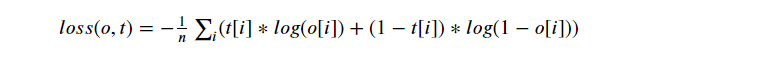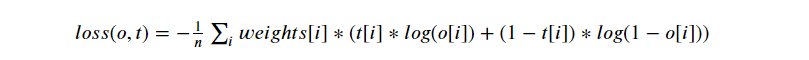# nn.L1Loss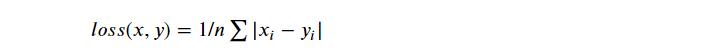## nn.NLLLoss

Negative Log Likelihood

``````class torch.nn.NLLLoss(weight=None, size_average=None, ignore_index=-100,
reduce=None, reduction='mean')
``````

``````loss(input, class) = -input[class]
``````

NLLLoss中如果传递了weight参数，会对损失进行加权，公式就变成了

``````loss(input, class) = -weight[class] * input[class]
``````

## nn.MSELoss

Mean Square Error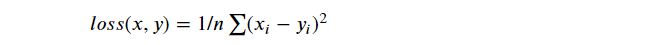## nn.CrossEntropyLoss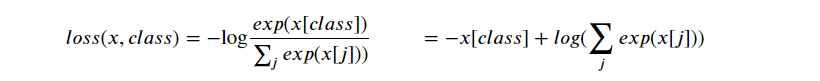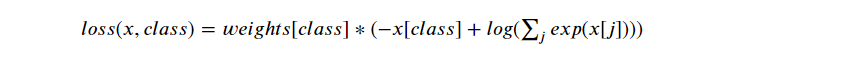## nn.BCELoss

Binary Cross Entropy